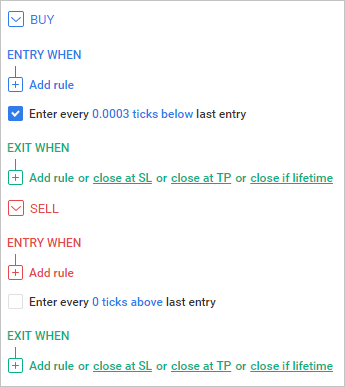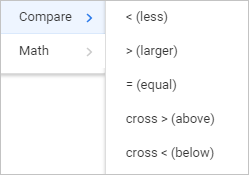It is very easy to set rules for opening and closing deals. Select the Editor tab on the top right corner of your window and expand the relevant area for Buy or Sell rules. Buy entering rules are marked blue, Sell entering rules are marked red. Exit rules are marked green and are used for both sides buy and sell deals.A rule is a condition based on different indicators. Every time the price changes it can be true or false. Find out more about indicators. You can create a variety of rules and connect them with logical operators: AND, OR.

In case of the "AND" operator both rules should be true to generate entry/exit signal.

In case of "OR" operator at least one rule should be true to generate entry/exit signal.

A rule always compares one value with the other. The value can be a result of calculated technical indicator or just a number (in case the indicator is Value). You can use basic math operations with two values and compare the result with another value.

Compare options:• < (less): True if the first value less than the second value
• > (larger): True if the first value larger than the second value
• = (equal): True if the first value equal to the second value
• Cross > (above): True only once after the first value becomes lager than the second value
• Cross < (below): True only once after the first value becomes less than the second value